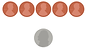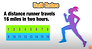### Math Warm-Ups# Would You Rather?# Clothesline Math# Challenging Math for Adventurous Learners# Math Problem of the Day

Expressions and Equations

Solve Me Mobiles

Number Sentence - Equations (Desmos)

The title doesn’t capture what this task is about.  It focuses on matching algebraic expressions to contexts and word descriptions.  It is a worthwhile task.

Equivalent Expressions (Desmos)

Pool Border Problem (Desmos)

Students explore different ways to find the number of tiles in the border of a square swimming pool.  They discover that the expressions they generate are all equivalent. (You would probably need to introduce this during a synchronous lesson and then have students work on it.)

Enter algebraic or numeric expressions and compare. **Really cool modeling!**

NCTM’s Algebra Tiles

Poses problems, has students model with algebra tiles and solve, tells them if they are correct or incorrect.

(PBS Media Learning)

Interactive opportunity to balance a scale.

Inequalities Polygraph (Desmos)

Video Game:  Dragon Box (algebra 12+)

Teaches all the rules for solving algebraic equations but does it visually in a virtual video game environment..

The App is \$7.99

Equality Explorer: Basics

Equality Explorer: Two Variables

Expression Exchange

Area Model AlgebraThe Number System

Ratios and Proportional ReasoningFunctions